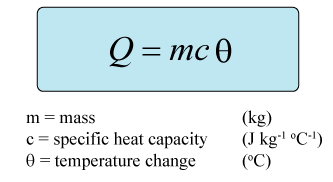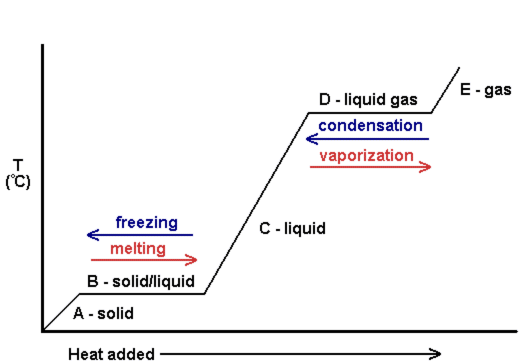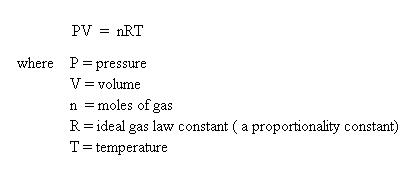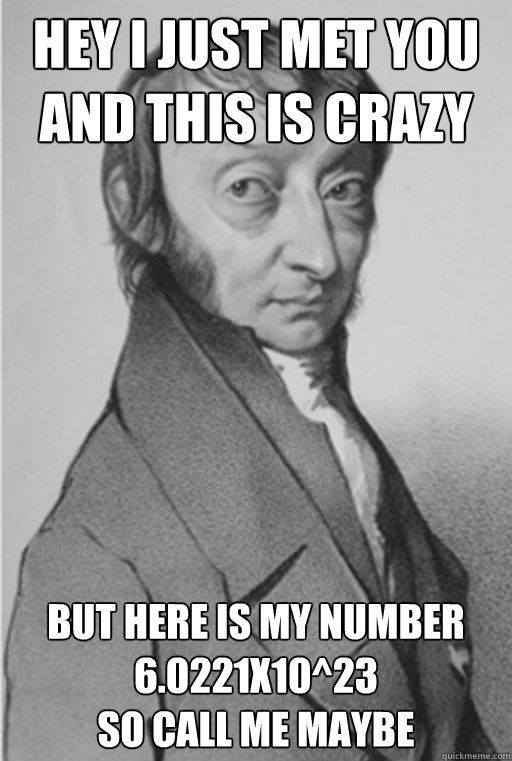# Topic 3: Thermal physics

See the guide for this topic.

## 3.1 – Thermal concepts

• ### Molecular theory of solids, liquids and gasesMolecules are held together by intermolecular forces.

The fourth state of plasma is not required to be learnt in the current syllabus.

• ### Temperature and absolute temperature

Temperatures describe how hot or cold an object is and determines the direction of heat flow between two bodies.

Thermal energy transfers from an object with higher temperature to another object with lower temperature. The energy transfer is called heat (the flow of energy due to temperature difference). Objects which are in thermal equilibrium have the same temperature.Temperatures in Kelvin can be calculated by deducting the temperatures in Celsius by 273.15.The absolute temperature of a body in the Kelvin scale is directly proportional to the average kinetic energy per molecule inside the body.

Absolute zero is 0K or -273 degrees Celsius.

Temperatures cannot be lower than absolute zero. It is the temperature where particles have zero average kinetic energy (no random motion).

• ### Internal energy

Internal energy is the sum of total kinetic energy (total thermal energy) and total potential energy.

Kinetic energy is energy associated with the random/translational rotational motions of molecules.

Potential energy is associated with forces between molecules.

• ### Specific heat capacity

The specific heat capacity of a substance is given byand is defined by the amount of heat needed to raise the temperature of 1kg of the substance by 1K.

Different substances have different specific heat capacities because of different densities and physical properties.

### FYI

The thermal capacity of an object is given by Q=cθ (or Q=cΔT) and is defined by the amount of heat needed to raise an object’s temperature by 1K.

• ### Phase change

 Change of phase Process Kinetic energy Potential energy Solid to liquid Melting Unchanged Increases Liquid to solid Freezing Unchanged Decreases Liquid to gas Boiling Unchanged Increases Gas to liquid Condensation Unchanged Decreases

During a phase change, temperature and kinetic energy remain constant and potential energy changes (which increases as molecules spread out and vice versa).

### FYI

Boiling: Only occurs at the boiling point (a specific temperature depending on substance) and occurs throughout the liquid.

Evaporation: Can occur at any temperature and only occurs at the surface of the liquid. The fastest moving particles evaporate, decreasing the average KE of the remaining particles. As a result, temperature drops.

• ### Specific latent heat

The specific latent heat of a substance is given bySpecific latent heat of fusion: The amount of heat required to change 1kg of a substance from solid to liquid without any change in temperature.

Specific latent heat of vaporization: The amount of heat required to change 1kg of a substance from liquid to gas without any change in temperature.## 3.2 – Modelling a gas

• ### Pressure

Pressure is defined as the normal force per unit area and is given by the equation• ### Equation of state for an ideal gaswhere R=8.31(J/mol/K)

• ### Kinetic model of an ideal gas

Assumptions:

• The collisions between molecules are perfectly elastic.
• The molecules are identical spheres.
• The volume of molecules is negligible compared to the volume of the gas.
• Molecules do not interact with each other except when they are in constant.

Implications:

Absolute temperature is directly proportional to the average KE and average speed of the molecules of an ideal gas.

• ### Mole, molar mass and the Avogadro constant

Mole:

• Like the word “dozon”, a mole is a unit of quantity. It is used to measure the number of atoms or molecules.
• A mole of any material contains 6.022*10^23 atoms or molecules. The value 6.022*10^23 is called the Avogadro constant.
• The number of moles of a substance can be calculated by dividing the number of molecules of that substance by the Avogadro constant.

Molar Mass:

• The molar mass is the mass of 1 mole of any element or compound.
• Different materials/elements have different molar masses which can be found as the Mr in the periodic table.

6.022*10^23• ### Differences between real and ideal gases

The ideal gas is based on a list of assumptions stated previously. However, in real gases, such assumptions may not be true.

• Forces exist between gas molecules in real gases (intermolecular forces).
• The volume of molecules is not negligible compared to the volume of gas in real gases.

Real gases may behave similarly to ideal gases under high temperatures and low pressure.